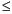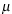Functions and CALL Routines

# CINV Function

Returns a quantile from the chi-square distribution.
 Category: Quantile

## Syntax

 CINV (p,df<,nc>)

### Arguments

p

is a numeric probability.

 Range: 0p < 1
df

is a numeric degrees of freedom parameter.

 Range: df > 0
nc

is a numeric noncentrality parameter.

 Range: nc0

The CINV function returns the pth quantile from the chi-square distribution with degrees of freedom df and a noncentrality parameter nc. The probability that an observation from a chi-square distribution is less than or equal to the returned quantile is p. This function accepts a noninteger degrees of freedom parameter df.

If the optional parameter nc is not specified or has the value 0, the quantile from the central chi-square distribution is returned. The noncentrality parameter nc is defined such that if X is a normal random variable with meanand variance 1, X2 has a noncentral chi-square distribution with df=1 and nc =2.

CAUTION:
For large values of nc, the algorithm could fail. In that case, a missing value is returned.Note:   CINV is the inverse of the PROBCHI function.The first statement following shows how to find the 95th percentile from a central chi-square distribution with 3 degrees of freedom. The second statement shows how to find the 95th percentile from a noncentral chi-square distribution with 3.5 degrees of freedom and a noncentrality parameter equal to 4.5.

SAS Statements Results
`q1=cinv(.95,3);`
`7.8147279033`
`a2=cinv(.95,3.5,4.5);`
`7.504582117`

 Functions:Previous Page | Next Page | Top of Page